# Mesh and nodal analysis

Aim

To analyse resistive-based circuits using Kirchhoff’s current and voltage laws (KCLs and KVLs).

To calculate voltages and currents based on a Matlab program.

Nodal analysis of resistive circuits

Using Kirchhoff’s current law (KCL), the nodal analysis is done by first naming the nodes and calculating the currents at the nodes (Bird, 2017). The nodes are named 1, 2, 3, 4 and 5 while the nodal voltages are named V1, V2, V3, V4 and V5 as shown in the figure below (Gussow, 2011).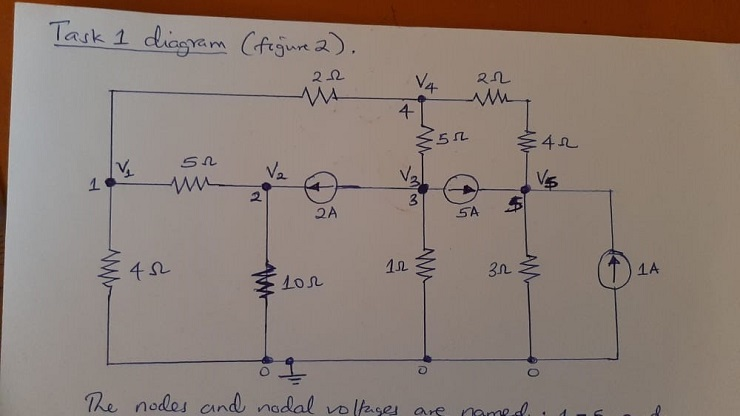KCL loop equations

Node 1:

V1/4+ (V1-V2)/5+(V1-V4)/2=0

Simplifies to

0.95 V1-0.2 V2-0.5 V4=0

Node 2:

(V2-V1)/5+V2/10-2=0

-0.2 V1+0.3 V2=2

Node 3:

(V3-V4)/5+V3/1+2+5=0

1.2 V3-0.2 V4=-7

Node 4:

(V4-V1)/2+(V4-V5)/((2+4))+(V4-V3)/5=0

-0.5 V1-0.2 V3+13/15 V4-1/6 V5=0

Node 5:

(V5-V4)/((2+4))+V5/3-5-1=0

-1/6 V4+0.5 V5=6

The equations are written in matrix form as: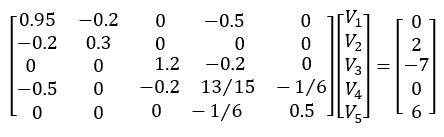MATLAB code

clc

clear

G1=[0.95 -0.2 0 -0.5 0; -0.2 0.3 0 0 0; 0 0 1.2 -0.2 0; -0.5 0 -0.2 ...

13/15 -1/6; 0 0 0 -1/6 0.5]; %Defining conductance matrix

I1=[0;2; -7; 0; 6]; %Defining the current matrix

G1_inv=inv(G1); %inverse of G1

disp("Task 1: Nodal Voltages from Nodal Analysis")

V1=G1_inv*I1 % Calculation of nodal voltages

Task 1: Nodal Voltages from Nodal Analysis

V1 =

3.7740

9.1827

-5.2504

3.4976

13.1659

Therefore,

V1=3.7740 V,V2=9.1827 V,V3=-5.2504 V, V4=3.4976 V,V5=13.1659

Mesh analysis of the resistive circuits

Using Kirchhoff’s voltage law, the circuit of Task 2 is analyzed as follows (B L Theraja et al., 2012):

Loop 1:

4i1+(i1-i2 )+3(i1-i3 )=0

8i1-i2-3i3=0

Loop 2:

-10+i2+2(i2-i4 )+(i2-i1 )=0

-i1+4i2-2i4=10

Loop 3:

5+2i3+3(i3-i1 )=0

-3i1+5i3=-5

Loop 4:

-5+2(i4-i2 )+5(i4-i5 )=0

-2i2+7i4-5i5=5

Loop 5:

1+2(i5-i6 )+5(i5-i4 )=0

-5i4+7i5-2i6=-1

Loop 6:

-3+2(i6-i5 )+5i6=0

-2i5+7i6=3

The equations are written in matrix form as: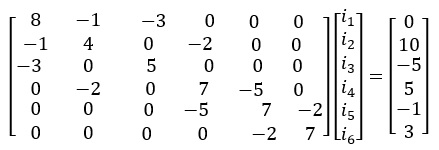MATLAB code

R2=[8 -1 -3 0 0 0; -1 4 0 -2 0 0; -3 0 5 0 0 0; 0 -2 0 7 -5 0; 0 0 0 -5 ...

7 -2; 0 0 0 0 -2 7]; %Define the resistance matrix

V2=[0;10; -5; 5; -1; 3]; %Define the voltage matrix

R2_inv=inv(R2); %Obtaining the inverse of resistance

disp("Task 2: Loop Currents from Mesh Analysis")

I2=R2_inv*V2 %Calculation of mesh currents

Task 2: Loop Currents from Mesh Analysis

I2 =

0.3159

4.9585

-0.8105

4.7590

3.6792

1.4798

Therefore:

i1=0.3159 A,i2=4.9585 A,i3=-0.8105 A

i4=4.7590 A,i5=3.6792 A,i6=1.4798 A

Textbook problem P2.57

Since the voltage at node 3, (V3) is known to be equal to the controlled voltage source, 2Vx, the number of nodes reduces to 2 (A Balakrishnan & T Vasantha Balakrishnan, 2018).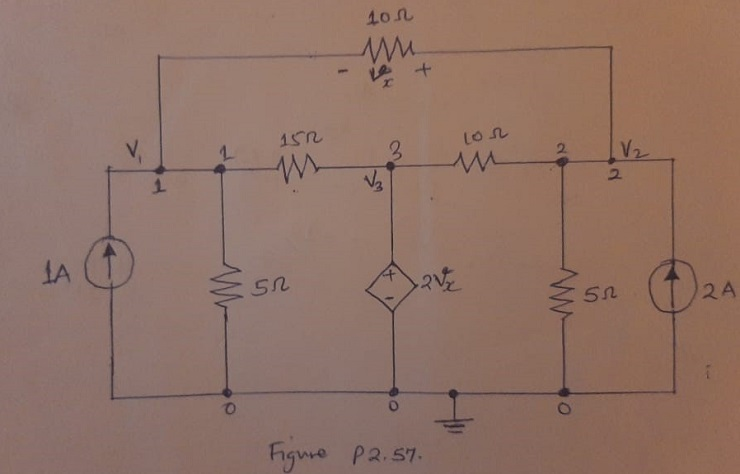Node 1: -1+(V1-V2)/10+(V1-V3)/15+V1/5=0

11/30 V1-0.1V2-1/15 V3=1

Node 2:

-2+(V2-V1)/10+(V2-V3)/10+V2/5=0

-0.1V1+0.4V2-0.1V3=2

At node 3, V3 is given by 2Vx Vx

=(V2-V1)/10

V3=(V2-V1)/5

Simplifying the nodal equations using expression for V3

11/30 V1-0.1V2-1/15×((V2-V1 ))/5 =1

19/50 V1-17/150 V2=1

-0.1V1+0.4V2-0.1×((V2-V1 ))/5=2

0.12V1+0.38V2=2

In matrix form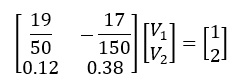MATLAB Code

## Textbook Q P2.57

G3=[19/50 -17/150; 0.12 0.38]; %Defining conductance matrixI3=[1;2]; %Defining the current matrixG3_inv=inv(G3); %inverse of G3disp("Task 3: Nodal Voltages for Figure P2.57")V3=G3_inv*I3 % Calculation of nodal voltages

Task 3: Nodal Voltages for Figure P2.57V3 = 3.8397 4.0506

Textbook P2.58

Creating a supernode as shown in the figure below, we do the nodal analysis (Hambley, 2019)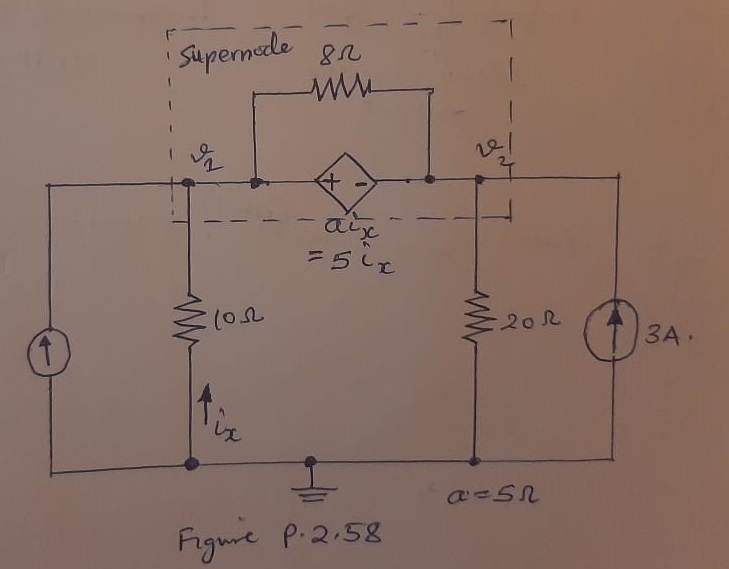We know that;

V1-V2=ai_x

a=5 Ω,i_x=V1/10

V1-V2=0.5V1

0.5V1-V2=0

Considering the supernode;

-5+V1/10+V2/20-3=0

0.1 V1+0.05V2=8

P=V^2/R=(ai_x )^2/R=(V1⁄10)^2/8=(V1-V2 )^2/8

In matrix form:

MATLAB Code

## Textbook Q P2.58

G4=[0.5 -1; 0.1 0.05]; %Defining conductance matrixI4=[0;8]; %Defining the current matrixG4_inv=inv(G4); %inverse of G4disp("Task 4: Nodal Voltages for Figure P2.58")V4=G4_inv*I4 % Calculation of nodal voltages% Power delivered to 8 ohms resistordisp("Power delivered to 8 Ohms resistor")P=((V4(1)-V4(2))^2)/8 %watts

Task 4: Nodal Voltages for Figure P2.58V4 =    64    32Power delivered to 8 Ohms resistorP =   128

ConclusionBy writing KCL equations, nodal analysis was done in task 1 to obtain the equations with unknown nodal voltages in matrix form. MATLAB program was then used to solve for the nodal voltages. By writing KVL equations, mesh analysis was done in task 2 to determine the loop currents by solving the resulting matrix equations for unknown currents. In Problem P2.57, nodal analysis was used to determine the nodal voltages in a similar way as task 1. Finally, in problem P2.58, nodal analysis was used to calculate the nodal voltages V1 and V2 which were then used to calculated power delivered to the 8 Ω resistor. The voltage across the 8 Ω resistor is the difference of the nodal voltages.

References

A Balakrishnan, & T Vasantha Balakrishnan. (2018). Electrical circuit theory. Ibs Buku.

Autor: B  L Theraja, Theraja, A. K., & Tarnekar, S. G. (2012). A textbook of electrical technology. Volume I, Basic electrical engineering : in S.I. system of units. S. Chand & Company Ltd.